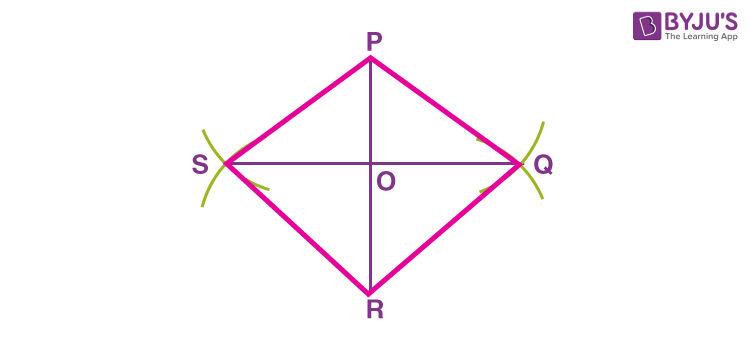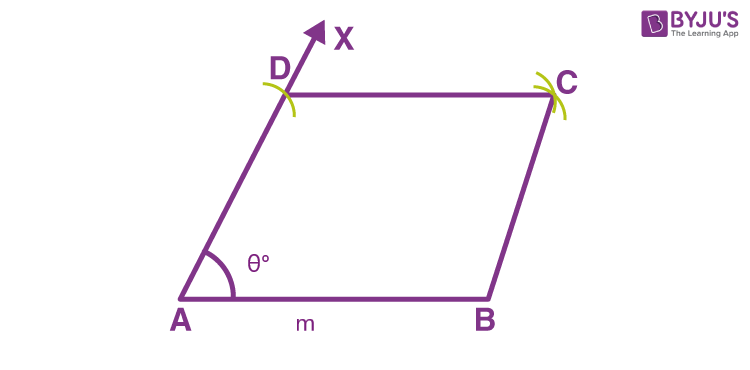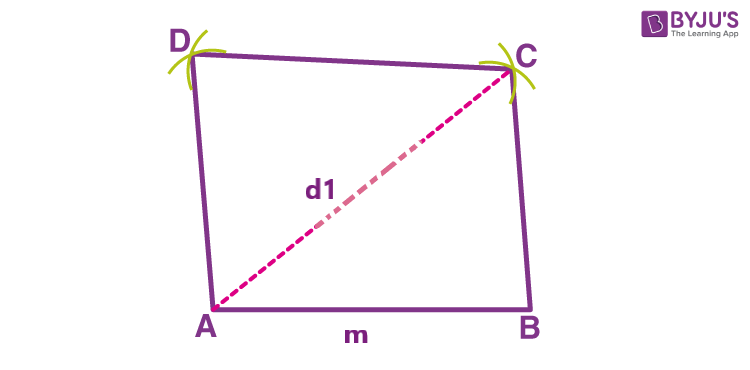Checkout JEE MAINS 2022 Question Paper Analysis : Checkout JEE MAINS 2022 Question Paper Analysis :

# Construction Of Rhombus

Construction of rhombus will explain here how to draw a rhombus with the given combination of measurements, such as:

• Length of its two diagonals
• Length of its side and measure of one angle
• Length of its side and one diagonal

Before talking about the construction of rhombus, let us recall the properties of a rhombus. A rhombus is a quadrilateral that has all its four sides equal in length. The diagonals of the rhombus bisect each other at a right angle. Also, the opposite angles of a rhombus are equal in measure and opposite sides are parallel to each other.

In this article, we will discuss steps to construct a rhombus, where the measurement of its two diagonals is given. Class 8 students can learn to construct a rhombus with the simple steps given here.

## Construction of Rhombus Given Length of Two Diagonals

A rhombus is a quadrilateral with sides of equal length. Opposite sides are parallel and opposite vertex angles are equal. Let us say, you are required to construct a rhombus PQRS with the lengths of its two diagonals, PR = 6 cm and SQ = 7 cm. We know that the diagonals of a rhombus bisect each other at 90 degrees. Hence, we do not require any other dimension for the construction of rhombus. The steps are:

Step 1: Draw a vertical line segment PR of length 6 cm.Step 2: Take the compass of any radius and from the point P, mark an arc towards the left and the other towards the right of PR. Repeat the same from point R without changing the compass radius.Step 3: The other diagonal measures 7 cm. Thus, draw a line joining the two points where the arc intersected each other in such a way that OS = OQ = 7/2 = 3.5 cmStep 4: Join the point P with Q, Q with R, R with S and S with P.PQRS is the required rhombus with the diagonals of lengths 6 cm and 7 cm. Note that O is the mid-point of the two diagonals and ∠POQ = ∠QOR = ∠ROS = ∠SOP = 90 degrees.

## Construction of Rhombus Given One Side and One Angle

If we are provided with one side and one angle of a rhombus, then follow the below steps to construct the rhombus.

• Draw the line segment of the given length, say AB
• At one endpoint of the line segment, draw an angle of a given measure, say ∠BAX
• With A as center and length equal to AB, draw an arc cutting the ray AX at D
• Now with D as center and radius equal to AB, draw an arc
• Taking B as center and with same radius, cut the previous arc at C
• Now join DC and BC

Hence, ABCD is the required rhombus.

An example figure is given below.## Construction of Rhombus Given one Diagonal and one Side

If we are given with length of one diagonal and one side, then we can construct a rhombus following the below steps:

• Draw a line segment AB equal to the length of the given side of the rhombus
• With A as center and radius equal to the length of diagonal draw an arc on the right side of point A and above B
• With B as center and radius equal to AB, cut the previous arc at C
• With C as center and radius equal to AB, draw an arc
• With A as center and same radius, cut the previous arc at D
• Join AD, BC and CD

Hence, ABCD is the required rhombus.

An example figure is given below.## Practice Questions

Here are some sample questions, students can practice drawing a rhombus.

1. Construct a rhombus with side PQ = 6 cm and angle P = 60 degrees
2. Construct a rhombus, with the length of one diagonal equal to 4.4 cm and another diagonal equal to 8 cm.

## Frequently Asked Questions – FAQs

### How to construct a rhombus?

A rhombus can be constructed if these details are available:
Length of its two diagonals or,
Length of its side and measure of one angle or,
Length of its side and one diagonal

### What are the properties of diagonal?

The sides of the diagonal are equal in length. The diagonals of a rhombus bisect each other at 90 degrees. The opposite sides of a rhombus are parallel and opposite angles are equal in measure.

### How to construct a rhombus when diagonals are given?

Draw the line segment equal to the length of the longest diagonal, say AB
Draw the perpendicular bisector of AB, that intersect AB at O
With O as center and radius equal to half of the length of another given diagonal, draw an arc on both sides of AC, to intersect the perpendicular bisector at B and D
Now join AB, BC, CD and AD
ABCD is the required rhombus